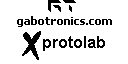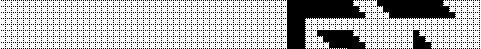Run Length Encoding for LCD

Bitmap Converter and Run Length Encoding for LCD

So you want to store one or a few bitmaps in your microcontroller but you find out that they need a lot of memory?
For example, a 128x64 bitmap will need 1KB of memory, that is a lot memory for low pin count micros. A simple solution: compress the image. Note that the decoding algorithm has to be simple, there is no point on having a complex algorithm that will take a lot of program memory anyway. The algorithm of choice for simple monochrome bitmaps is Run Length Encoding (RLE). Like the name suggests, Run Length Encoding compresses the data by replacing runs of the same data with a counter.

There are many variations, but the algorithm used is original from Arturo San Emeterio Campos:

 Encoder Decoder Get two bytesLoopAre they equal?Yes:    Output both of them    Count how many bytes repeated we have    Output that value    Update pointer to the input file    Get next two bytes    Repeat.No:    Output the first byte     Put the second, as first    Get a byte for the second one    Update pointer to input file    Repeat Get one byte, put it to the output file, and now it's the 'last' byteLoopGet one byteIs the current byte equal to last?Yes:    Now get another byte, this is 'counter'    Put current byte in the output file    Copy 'counter' times the 'last' byte to the output file    Put last copied byte in 'last' (or leave it alone)    RepeatNo:    Put current byte to the output file    Now 'last' is the current byte    Repeat

Let's apply the algorithm to the following image:As can be seen, there is a lot of white space, let's zoom in to see how the first few bytes are encoded. (The image will be used with a SSD1306. so the data is stored in vertical bytes).The encoded data is: '\x00','\x00','\x30','\xff','\xff','\x03','\x07','\x07','\x02','\x27','\x67','\xe7','\xe7','\x04','\xc6','\x84', .......

Let's interpret this data: two repeated numbers indicate a sequence, so 0x00, 0x00, 0x30 means that 0x00 is repeated 0x30 times.
Then there is 0xff, 0xff, 0x03, which means to repeat 0xff 0x03 times. Similarly, there is 0x07, 0x07, 0x02, which means to repeat 0x07 0x02 times.
Then there is 0x27, since there is only one, we place 0x27 once...

The encoding of the image can be done with this Bitmap Converter:And here are different decoding examples:

Code extract from the Xmegalab:

/* SED1335 example (Horizontal data orientation). Image size included in table */
void bitmap(uint8_t x, uint8_t y, const uint8_t *BMP) {
uint8_t u8Page=0;
uint8_t u8Column=0;
uint8_t data=0,count=0,low,high,width,height;
for (u8Page = y; u8Page < (y+height); u8Page++) { /* loop on the 8 pages */
Address = (u8Page<<4)+x; /* optimized for 128x128 display */
lcd_write_command(CSRW); /* Set cursor address */
lcd_write_data(low);
lcd_write_data(high); lcd_write_command(MWRITE);
for (u8Column=0; u8Column < width; u8Column++) {
if(count==0) {
}
else {
count = 1; i--;
}
}
count--;
lcd_write_data(data);
}
}
}

Code extract from the Xprotolab:

/* SSD1306 example (Vertical data orientation). Image size is fixed to 128 x 64 */
void GLCD_DisplayPicture (const uint8_t *pointer) {
uint8_t data=0, count=0;
uint16_t i;
uint8_t *p;
p = display_buffer;
for (i = 0; i < 1024; i++) {
if(count==0) {